## Insights at Disparate Scales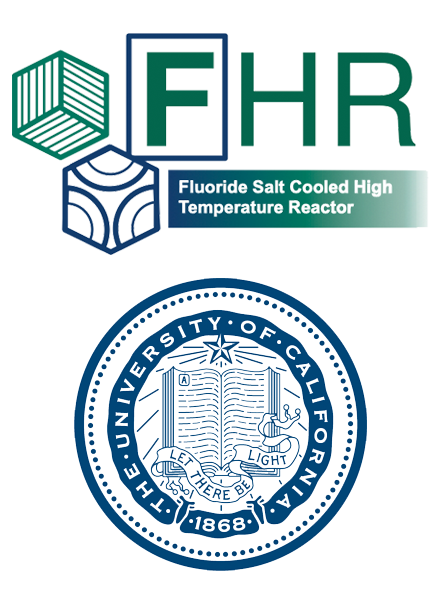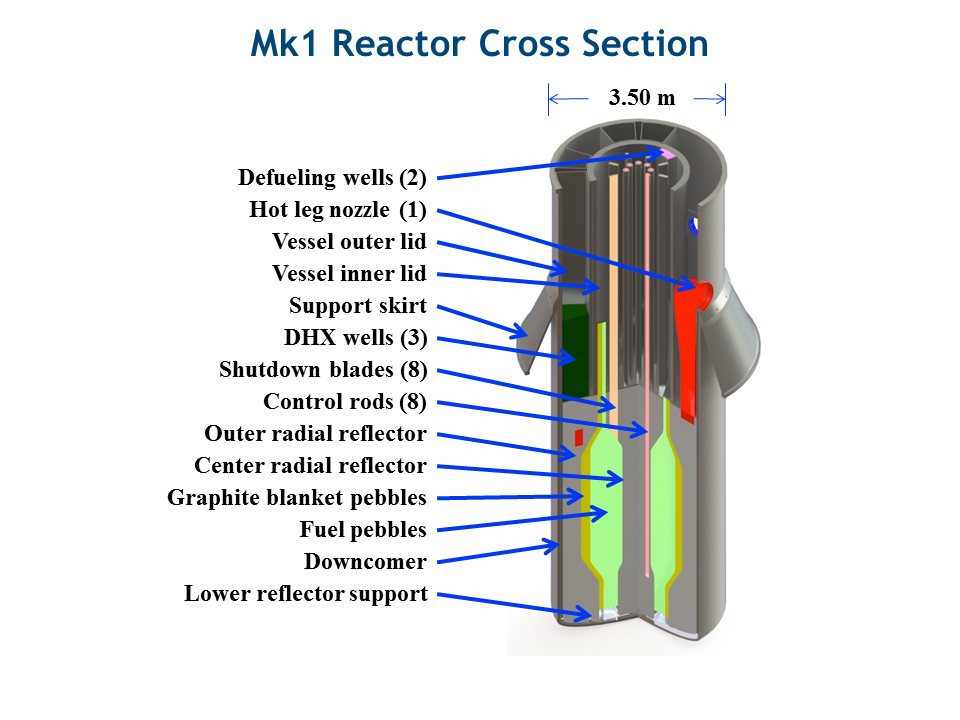## PB-FHR Fuel Geometry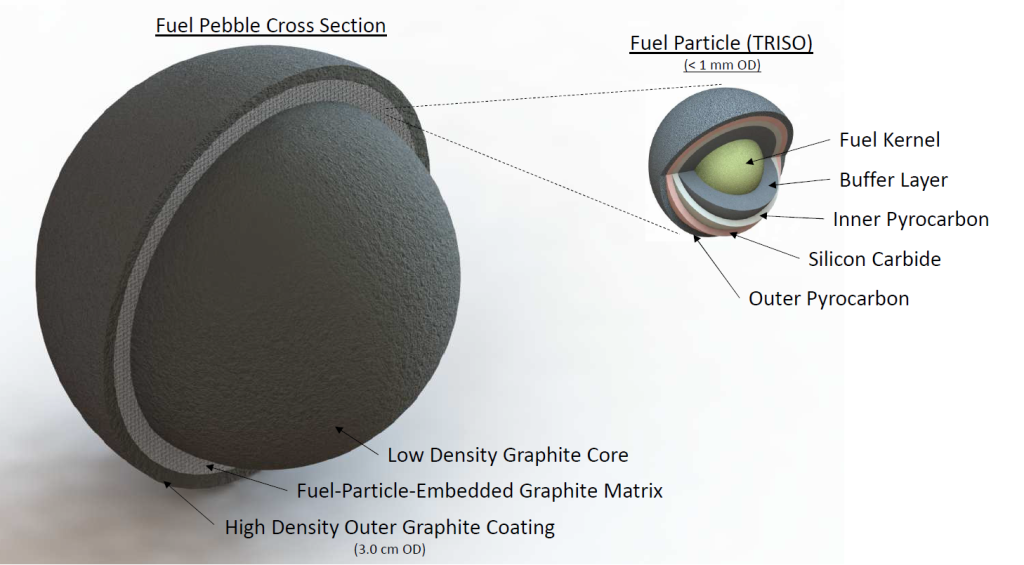## Coupled Multi-Physics Analysis

Severe accident neutronics and thermal hydraulics can be simulated beautifully for simple geometries and well studied materials. (below, INL BISON work.)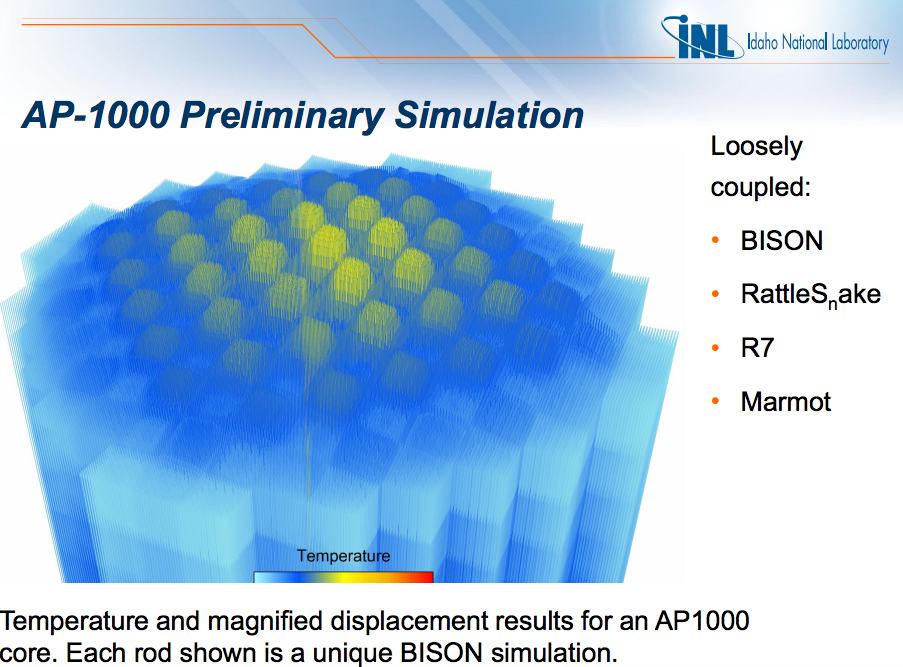## FHR, Accident Transient Analysis

• Collect experimental data
• Conduct algebraic, static, and benchmark results
• Develop 0D coupled neutronics/TH model (PyRK)
• Develop 3D neutronics/TH model
• Compare 0D and 3D models
• Couple additional physics (e.g. fuel performance)PyRK: Python for Reactor Kinetics

## Review of Nuclear Reactor Kinetics$\sigma(E,\vec{r},\hat{\Omega},T,x,i)$$k=1$

## Reactivity

\begin{align} k &= \mbox{"neutron multiplication factor"}\\ &= \frac{\mbox{neutrons causing fission}}{\mbox{neutrons produced by fission}}\\ \rho &= \frac{k-1}{k}\\ \rho &= \mbox{reactivity}\\ \end{align}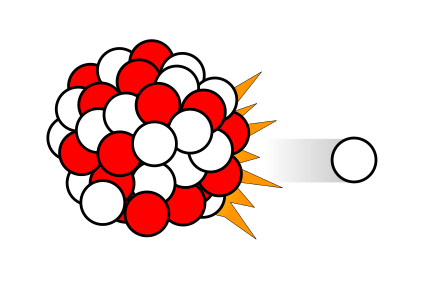$\beta_i, \lambda_{d,i}$

## PyRK

• 6-precursor-group,
• 11-decay-group Point Reactor Kinetics model
• Lumped Parameter thermal hydraulics model
• Object-oriented, geometry and material agnostic framework

## Point Reactor Kinetics

\begin{align} p &= \mbox{ reactor power }\\ \rho(t,&T_{fuel},T_{cool},T_{mod}, T_{refl}) = \mbox{ reactivity}\\ \beta &= \mbox{ fraction of neutrons that are delayed}\\ \beta_j &= \mbox{ fraction of delayed neutrons from precursor group j}\\ \zeta_j &= \mbox{ concentration of precursors of group j}\\ \lambda_{d,j} &= \mbox{ decay constant of precursor group j}\\ \Lambda &= \mbox{ mean generation time }\\ \omega_k &= \mbox{ decay heat from FP group k}\\ \kappa_k &= \mbox{ heat per fission for decay FP group k}\\ \lambda_{FP,k} &= \mbox{ decay constant for decay FP group k}\\ T_i &= \mbox{ temperature of component i} \end{align}
$\frac{d}{dt}\left[ \begin{array}{c} p\\ \zeta_1\\ .\\ \zeta_j\\ .\\ \zeta_J\\ \omega_1\\ .\\ \omega_k\\ .\\ \omega_K\\ T_{i}\\ .\\ T_{I}\\ \end{array} \right] = \left[ \begin{array}{ c } \frac{\rho(t,T_{i},\cdots)-\beta}{\Lambda}p + \displaystyle\sum^{j=J}_{j=1}\lambda_{d,j}\zeta_j\\ \frac{\beta_1}{\Lambda} p - \lambda_{d,1}\zeta_1\\ .\\ \frac{\beta_j}{\Lambda}p-\lambda_{d,j}\zeta_j\\ .\\ \frac{\beta_J}{\Lambda}p-\lambda_{d,J}\zeta_J\\ \kappa_1p - \lambda_{FP,1}\omega_1\\ .\\ \kappa_kp - \lambda_{FP,k}\omega_k\\ .\\ \kappa_{k p} - \lambda_{FP,k}\omega_{k}\\ f_{i}(p, C_{p,i}, T_{i}, \cdots)\\ .\\ f_{I}(p, C_{p,I}, T_{I}, \cdots)\\ \end{array} \right]$

## Lumped Parameter Heat Transfer

The heat flow out of body $i$ is the sum of surface heat flow by conduction, convection, radiation, and other mechanisms to each adjacent body, $j$: \begin{align} Q &= Q_i + \sum_j Q_{ij}\\ &=Q_i + \sum_j\frac{T_{i} - T_{j}}{R_{th,ij}}\\ \dot{Q} &= \mbox{total heat flow out of body i }[J\cdot s^{-1}]\\ Q_i &= \mbox{other heat transfer, a constant }[J\cdot s^{-1}]\\ T_i &= \mbox{temperature of body i }[K]\\ T_j &= \mbox{temperature of body j }[K]\\ j &= \mbox{adjacent bodies }[-]\\ R_{th} &= \mbox{thermal resistence of the component }[K \cdot s \cdot J^{-1}]. \end{align}

## Unit Checking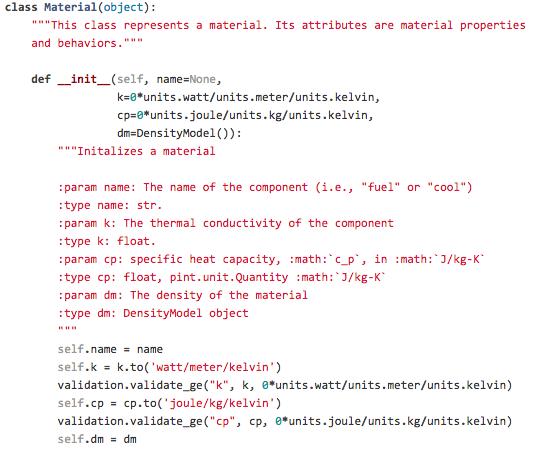In PyRK, the Pint package (pint.readthedocs.org/en/0.6/) is used keeping track of units, converting between them, and throwing errors when unit conversions are not sane.

## Version Control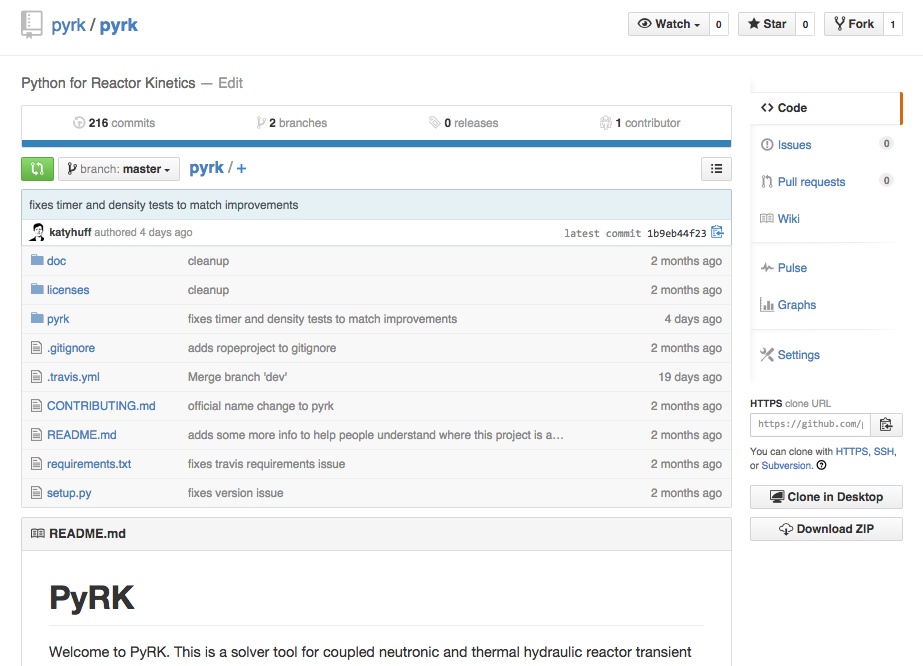Keeping track of versions of the code makes it possible to experiment without fear and placing the code online encourages use and collaboration.

## Automated Documentation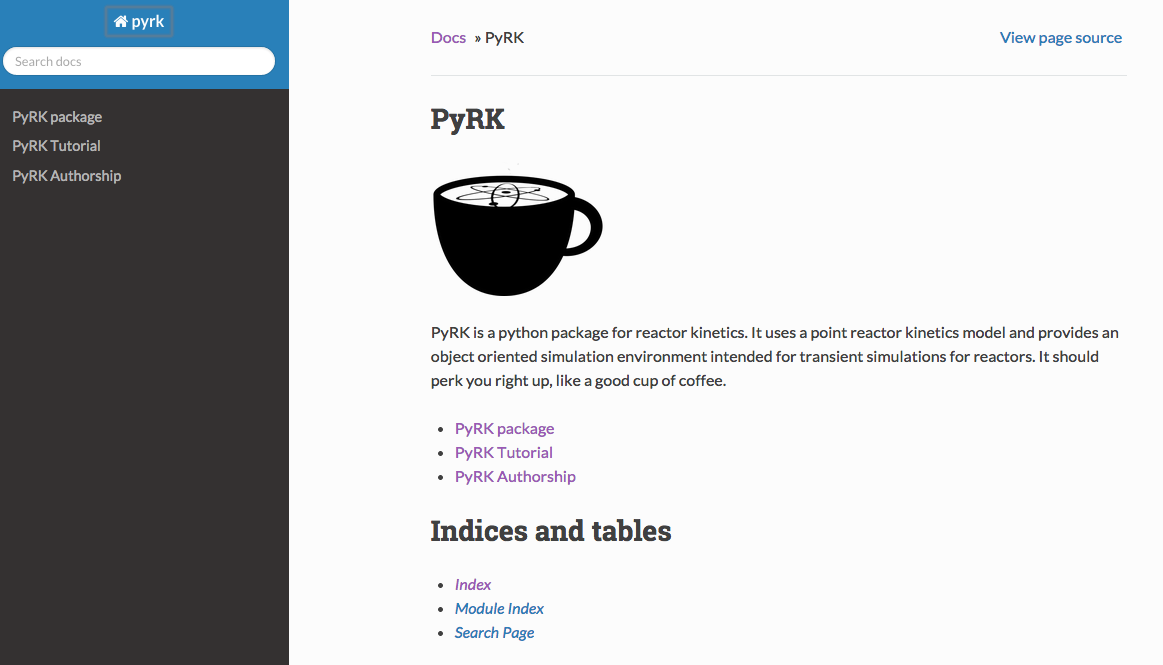Automated documentation creates a browsable website explaining the most recent version of the code.

## Test Suite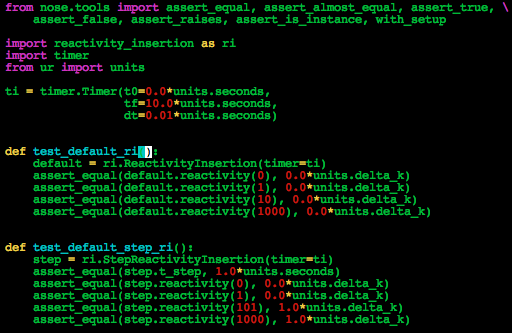The classes and functions that make up the code are tested individually for robustness using nose.

## Continuous Integration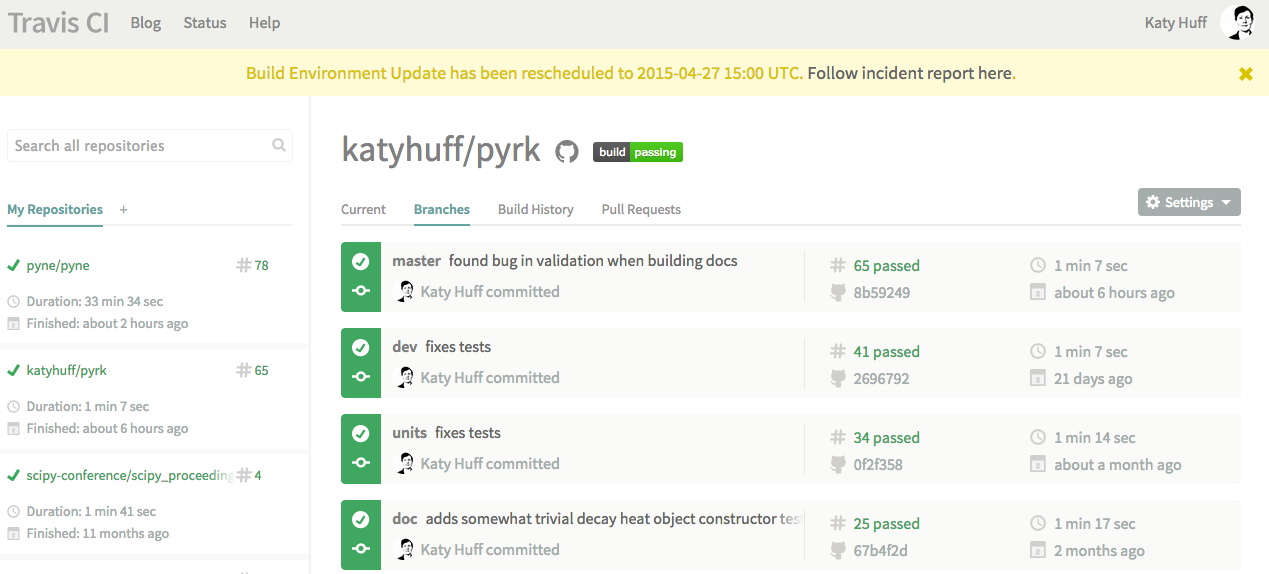The tests are run every time a change is made to the repository online. The results are public. If a main branch has a failed test, I get an email.

### PB-FHR Reactivity Insertion

Pebble-Bed, Fluoride Salt Cooled, High-Temperature Reactor
• Molten FLiBe Coolant
• Annular Core
• Annular Pebble Fuel
• Ramp Reactivity Insertion, 600pcm over 10s

### Ramp Reactivity Insertion


# External Reactivity
from reactivity_insertion import RampReactivityInsertion
rho_ext = RampReactivityInsertion(timer=ti,
t_start=t_feedback + 10.0*units.seconds,
t_end=t_feedback + 20.0*units.seconds,
rho_init=0.0*units.delta_k,
rho_rise=600.0*units.pcm,
rho_final=600.0*units.pcm)


### Components



fuel = th.THComponent(name="fuel",
mat=TRISO(),
vol=vol_fuel,
T0=t_fuel,
alpha_temp=alpha_fuel,
timer=ti,
heatgen=True,
power_tot=power_tot/n_pebbles,
sph=True,
ri=r_mod,
ro=r_fuel
)

mod = th.THComponent(name="mod",
mat=Graphite(),
vol=vol_mod,
T0=t_mod,
alpha_temp=alpha_mod,
timer=ti,
sph=True,
ri=0.0,
ro=r_mod)

cool = th.THComponent(name="cool",
mat=Flibe(),
vol=vol_cool,
T0=t_cool,
alpha_temp=alpha_cool,
timer=ti)

shell = th.THComponent(name="shell",
mat=Graphite(),
vol=vol_shell,
T0=t_shell,
alpha_temp=alpha_shell,
timer=ti,
sph=True,
ri=r_fuel,
ro=r_shell)


### Heat Transfer


# The coolant convects to the pebbles


### Ramp Reactivity Insertion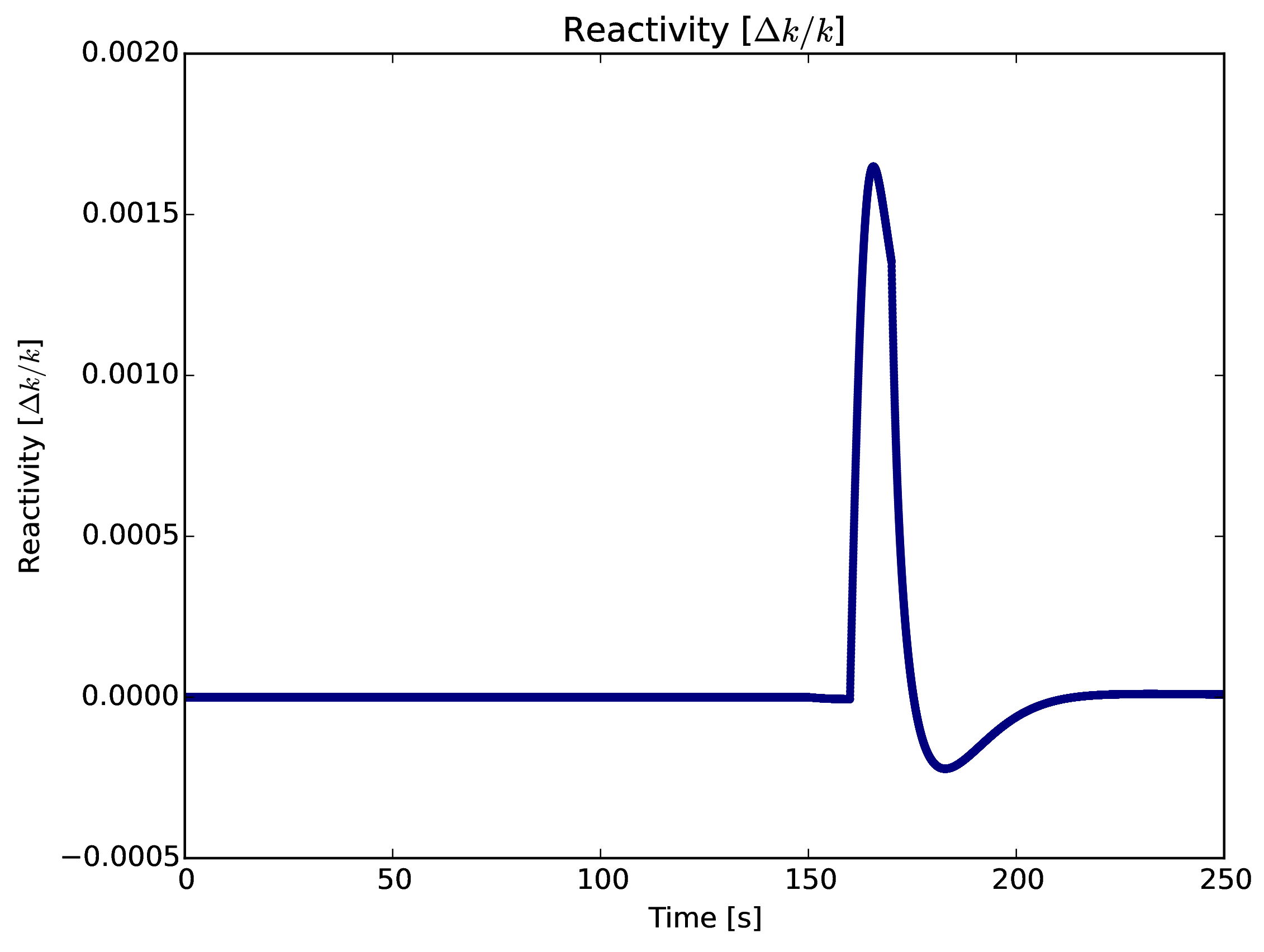### Pebble TemperatureAverage fuel pebble peak temperature $<1100^\circ C$## The Nuclear Fuel Cycle

Hundreds of discrete facilities mine, mill, convert, fabricate, transmute, recycle, and store nuclear material.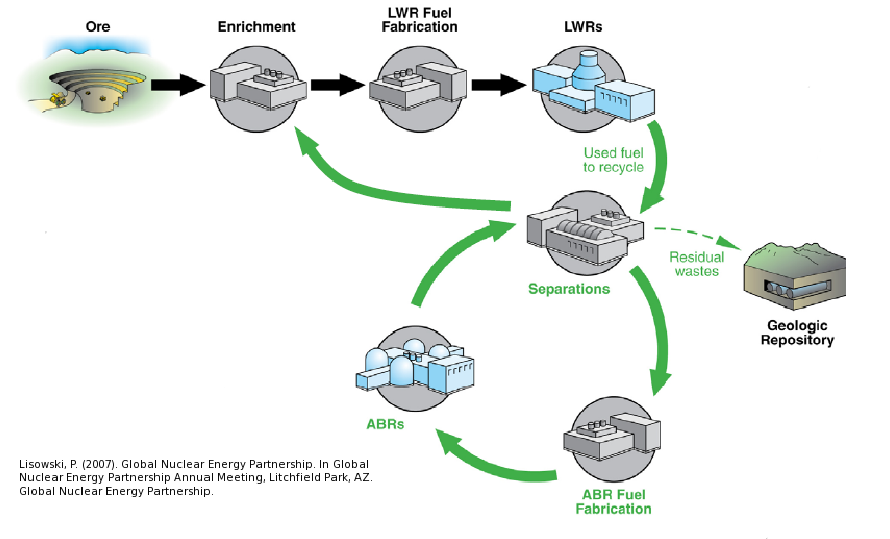## Fuel Cycle Metrics

• Mass Flow
• proliferation resistance and physical protection (PRPP) indices.
• Cost
• levelized cost of electricity,
• facility life cycle costs.
• Economics
• power production, facility deployments,
• dynamic pricing and feedback.
• Disruptions
• reliability, safety,
• system robustness.

### Current Simulators

• CAFCA (MIT)
• COSI (CEA)
• DANESS (ANL)
• DESAE (Rosatom)
• Evolcode (CIEMAT)
• FAMILY (IAEA)
• GENIUSv1 (INL)
• GENIUS v2 (UW)
• NFCSim (LANL)
• NFCSS (IAEA)
• NUWASTE (NWTRB)
• ORION (NNL)
• MARKAL (BNL)
• VISION (INL)

## State of the Art

### Performance

• Speed interactive time scales
• Fidelity: detail commensurate with existing challenges
• Detail: discrete material and agent tracking
• Regional Modeling: enabling international socio-economics

## Beyond the State of the Art

### Access

• Openness: for collaboration, validation, and code sustainability.
• Usability: for a wide range of user sophistication

### Extensibility

• Modularity: core infrastructure independent of proprietary or sensitive data and models
• Flexibility with a focus on robustness for myriad potential developer extensions.

## Extensibility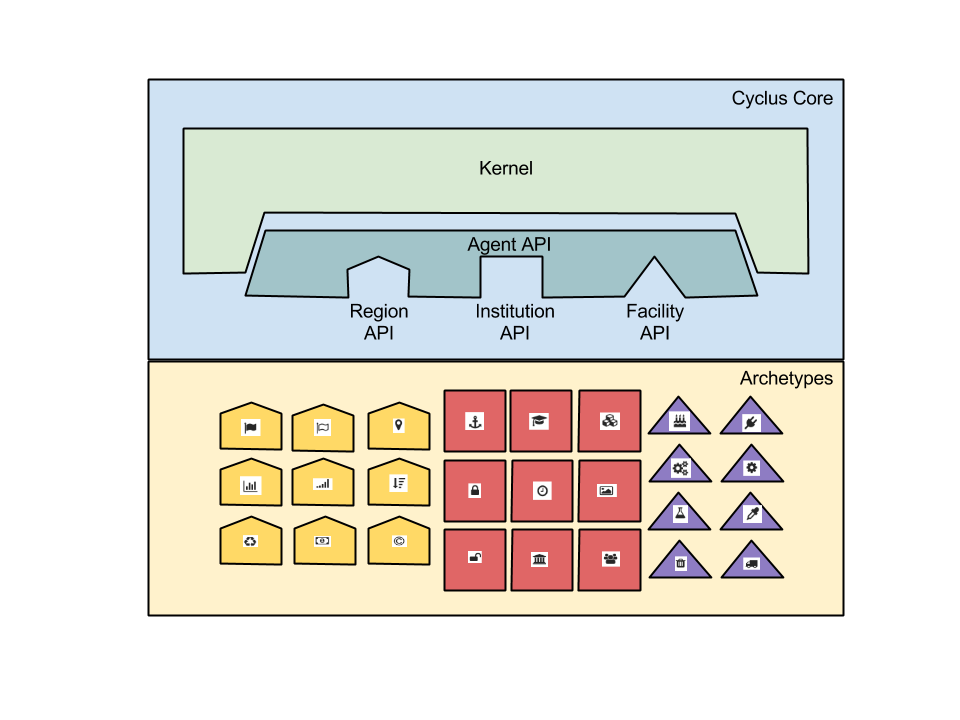## Openness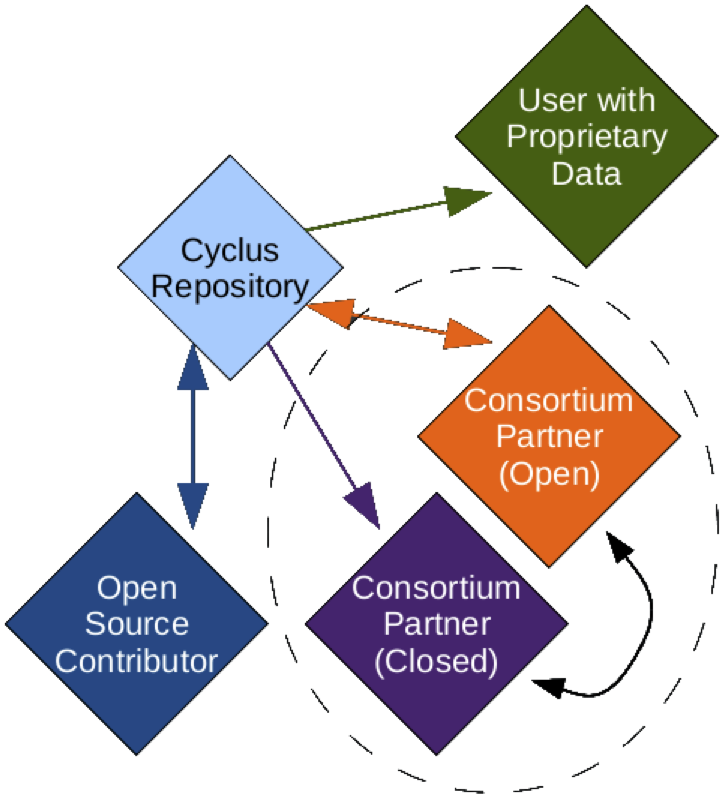## Growing Ecosystem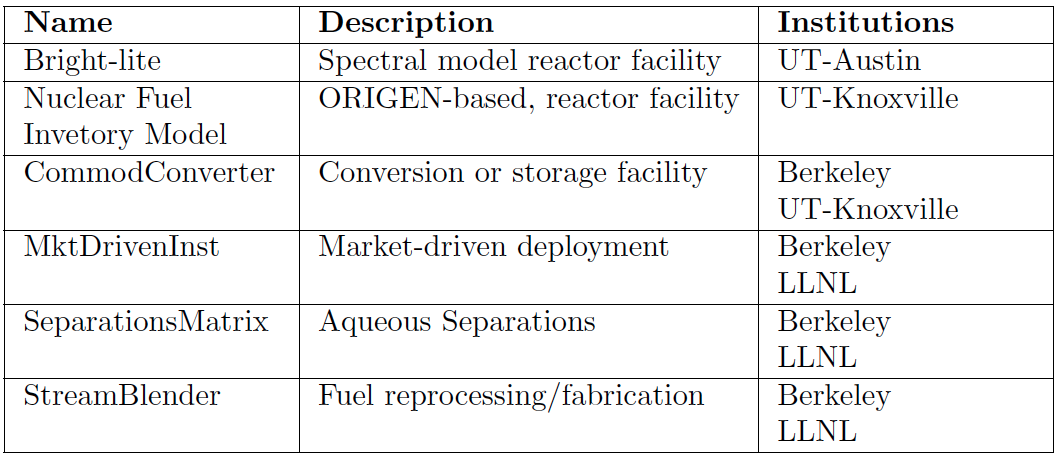## ...Well Beyond

### Algorithmic Sophistication

• Efficient: memory-efficient isotope tracking
• Customizable: constrained fuel supply
• Dynamic: isotopic-quality-based resource routing
• Physics-based: fuel fungibility

## Agent Based Systems Analysis

An agent-based simulation is made up of actors and communications between those actors.## Agent Based Systems Analysis

A facility might create material.## Agent Based Systems Analysis

It might request material.## Agent Based Systems Analysis

It might do both.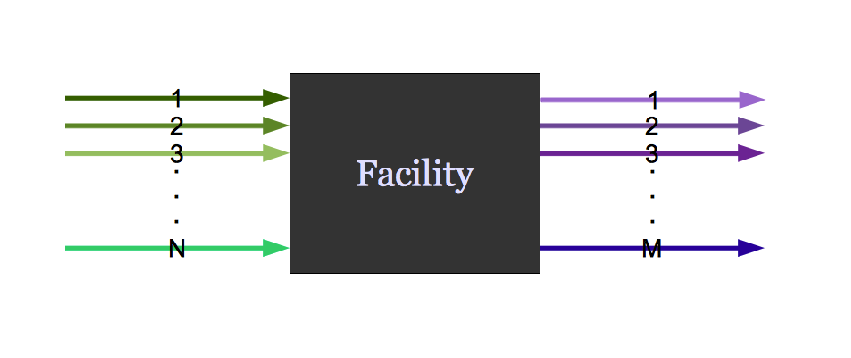## Agent Based Systems Analysis

Even simple fuel cycles have many independent agents.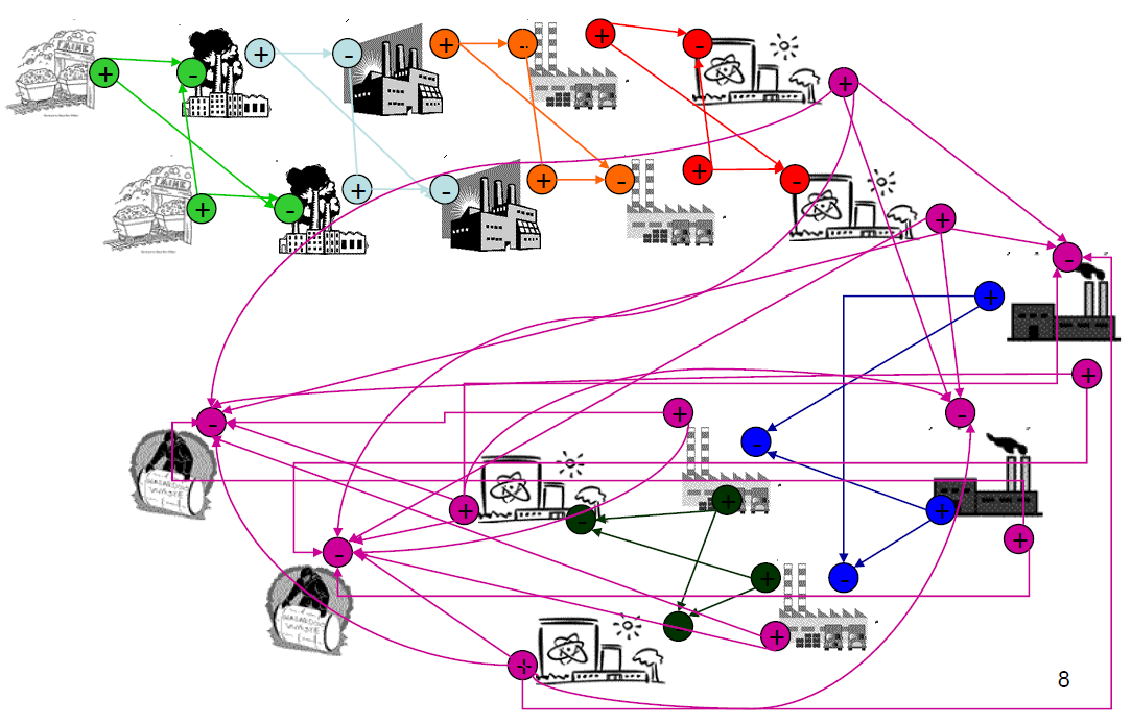## Agent Based Systems Analysis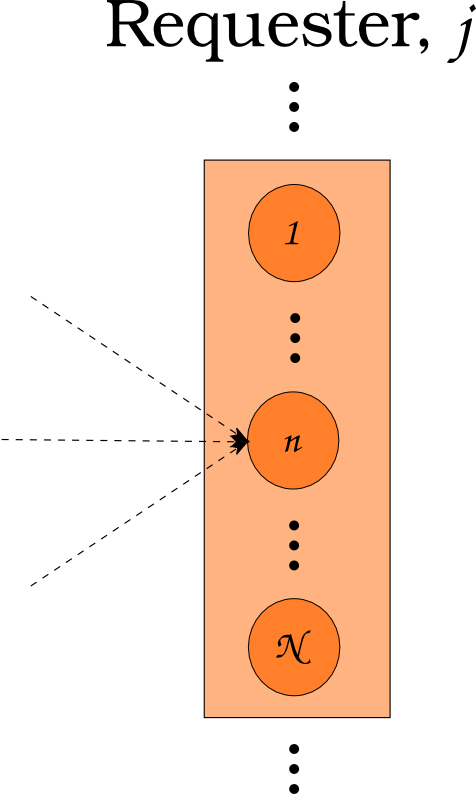$N_i \subset N$

## Agent Based Systems Analysis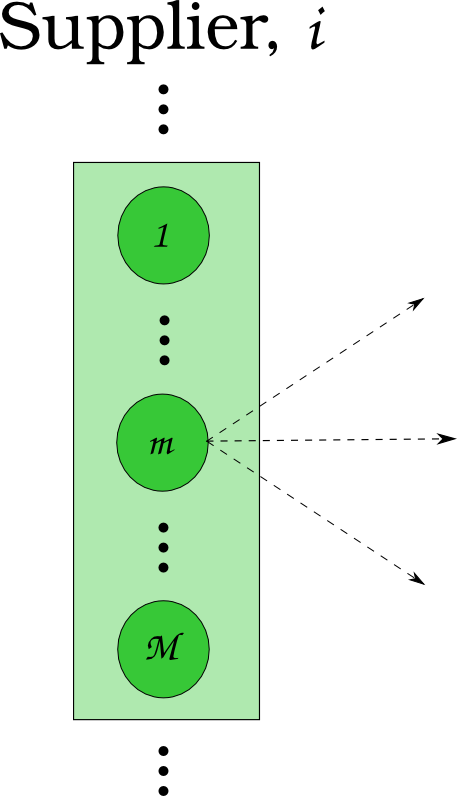$N_j \subset N$

## Agent Based Systems Analysis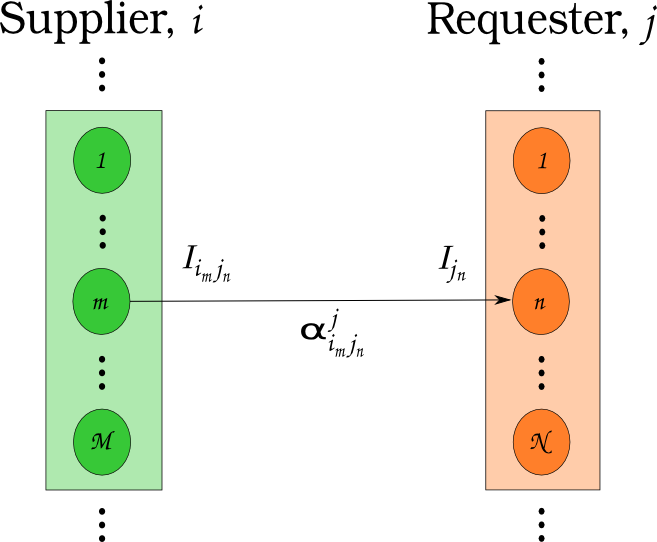$N_i \cup N_j = N$

## Feasibility vs. Optimization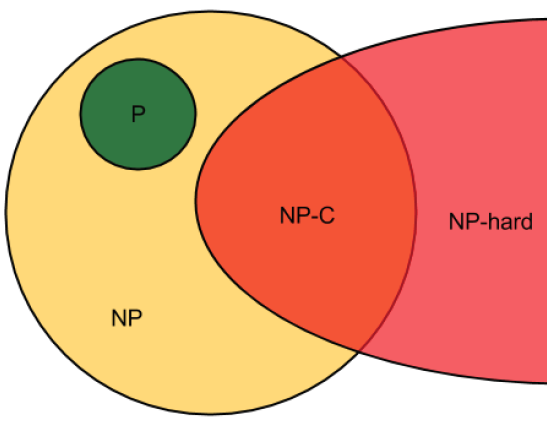If a decision problem is in NP-C, then the corresponding optimization problem is NP-hard.

## Multi-Commodity Transportation Formulation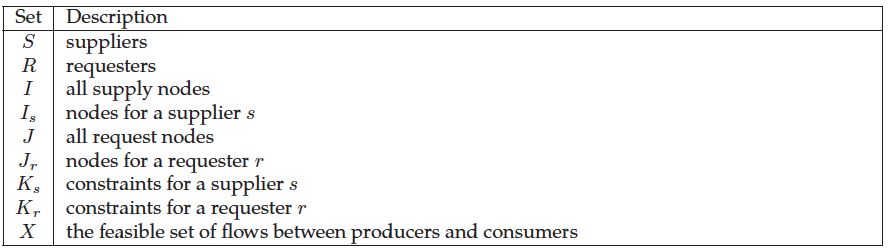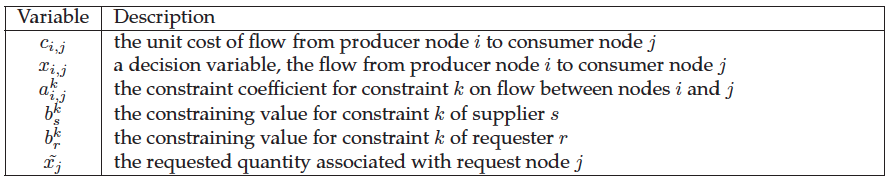## Multi-Commodity Transportation Formulation

\begin{align} \min_{x} z &= \sum_{i\in I}\sum_{j\in J} c_{i,j}x_{i,j} & \\ s.t & \sum_{i\in I_s}\sum_{j\in J} a_{i,j}^k x_{i,j} \le b_s^k & \forall k\in K_s, \forall s\in S\\ & \sum_{J\in J_r}\sum_{i\in I} a_{i,j}^k x_{i,j} \le b_r^k & \forall k\in K_r, \forall r\in R\\ & x_{i,j} \in [0,x_j] & \forall i\in I, \forall j\in J \end{align}

## Dynamic Resource Exchange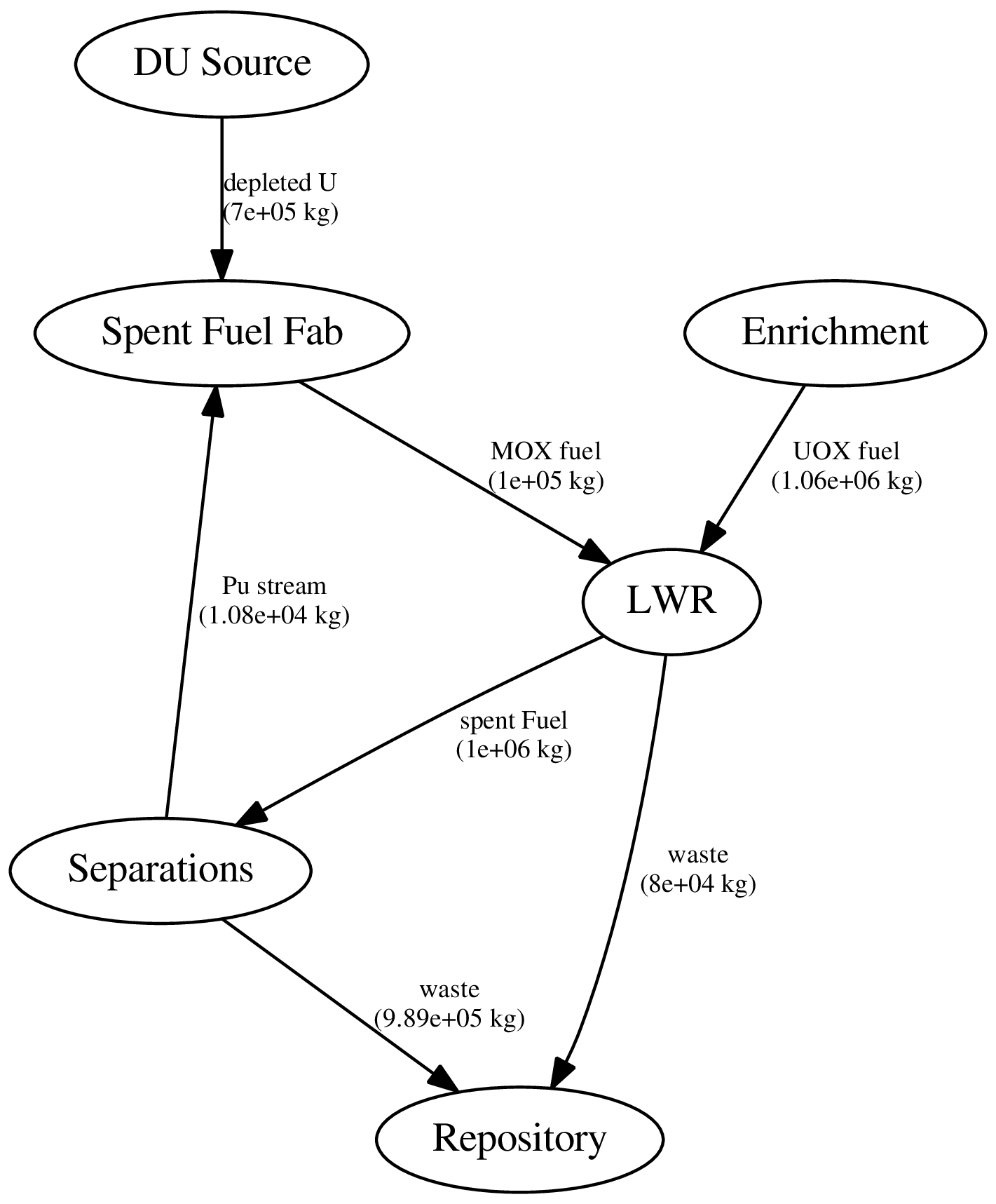## Dynamic Resource Exchange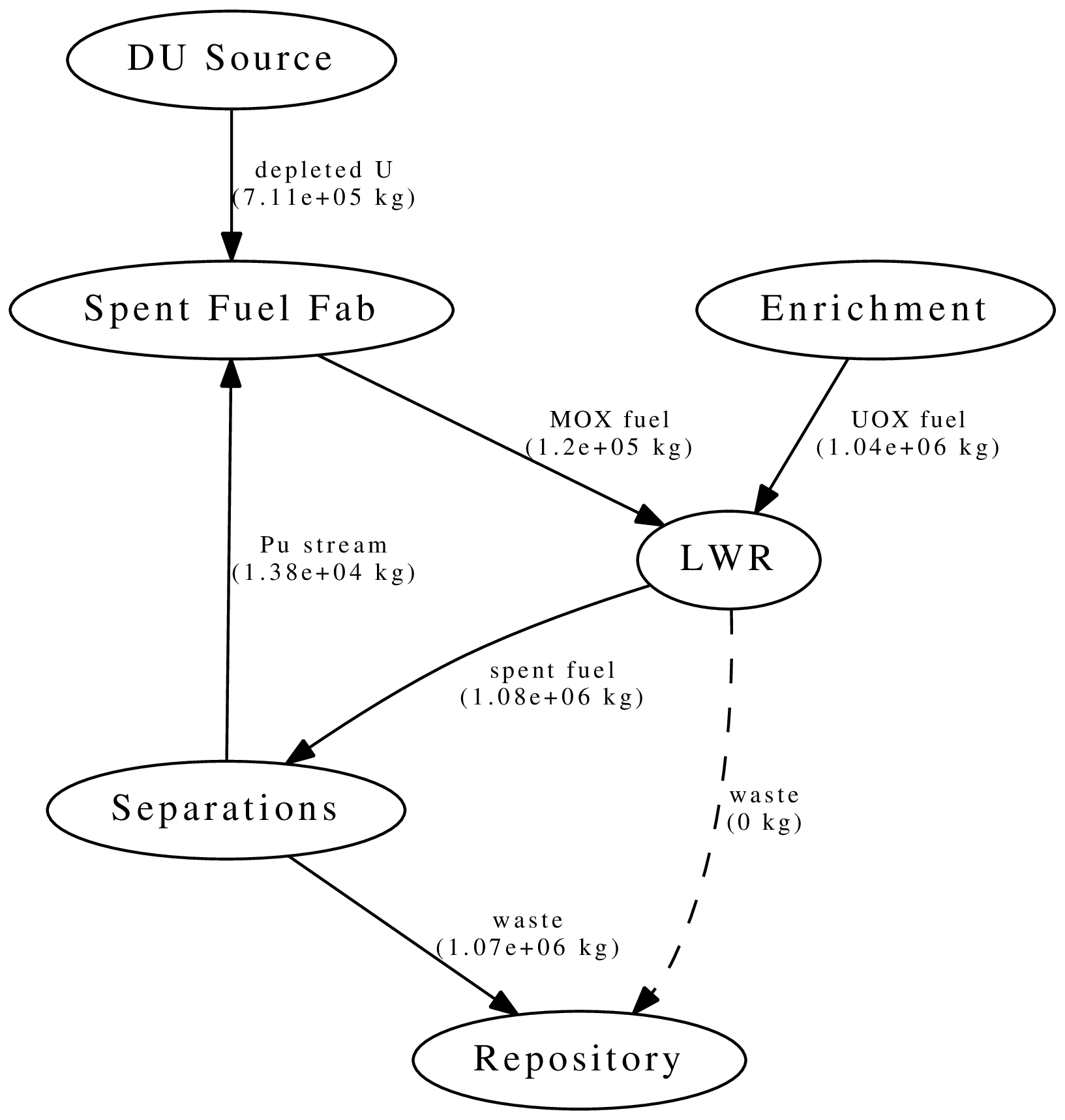## Dynamic Resource Exchange



mox
-    waste
+    spent_fuel
mox_fresh_fuel
mox_spent_fuel



## Dynamic Resource Exchange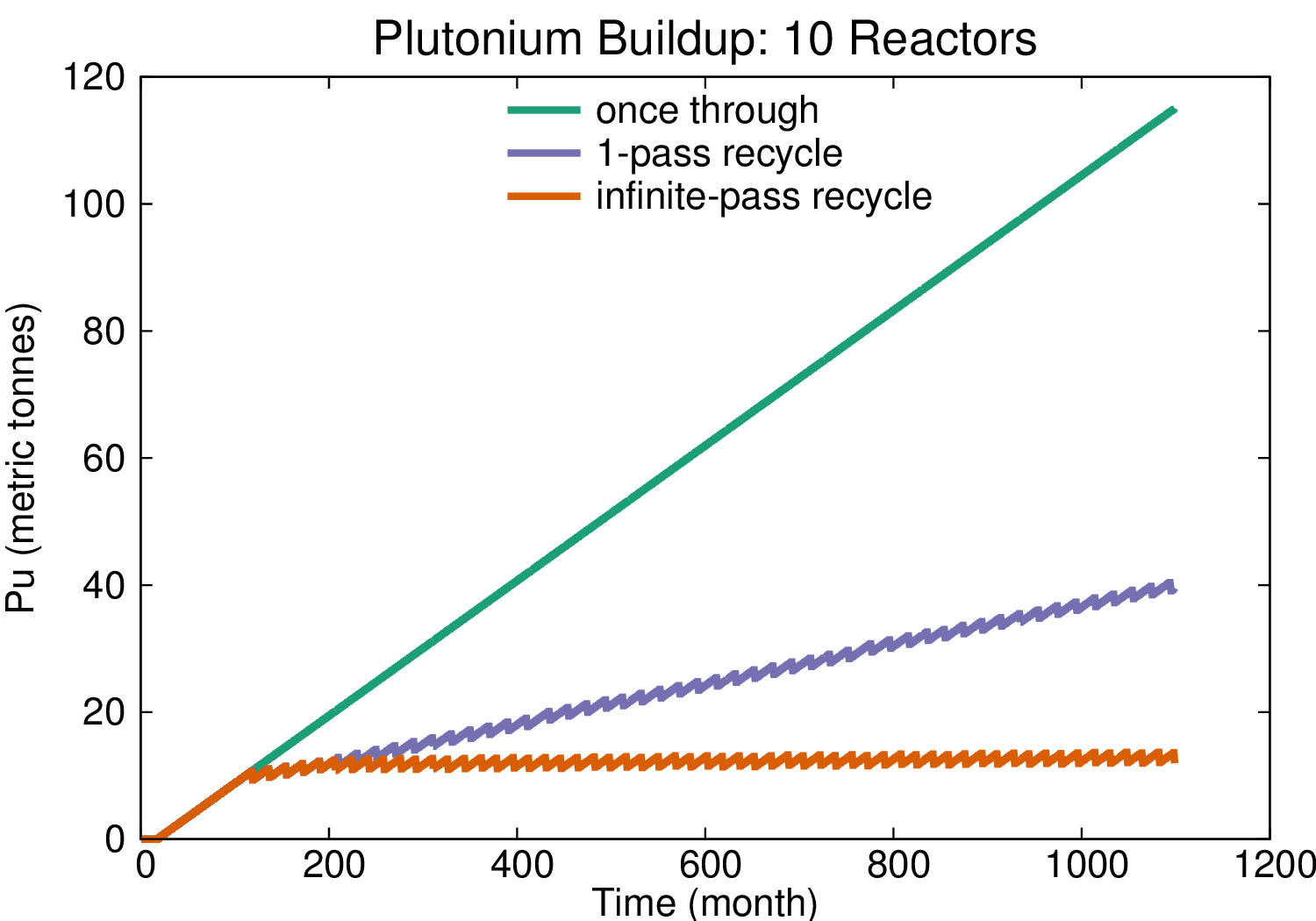## Transition Analysis

• LWR to SFR
• $T_0 = 2015$
• $T_f <= 2215$
• $C_0 = 100$ GWe LWR
• Annual nuclear energy demand growth: 1%
• Spent LWR fuel reprocessed to fabricate FR fuel
• Spent FR fuel reprocessed to fabricate FR fuel

## Transition Analysis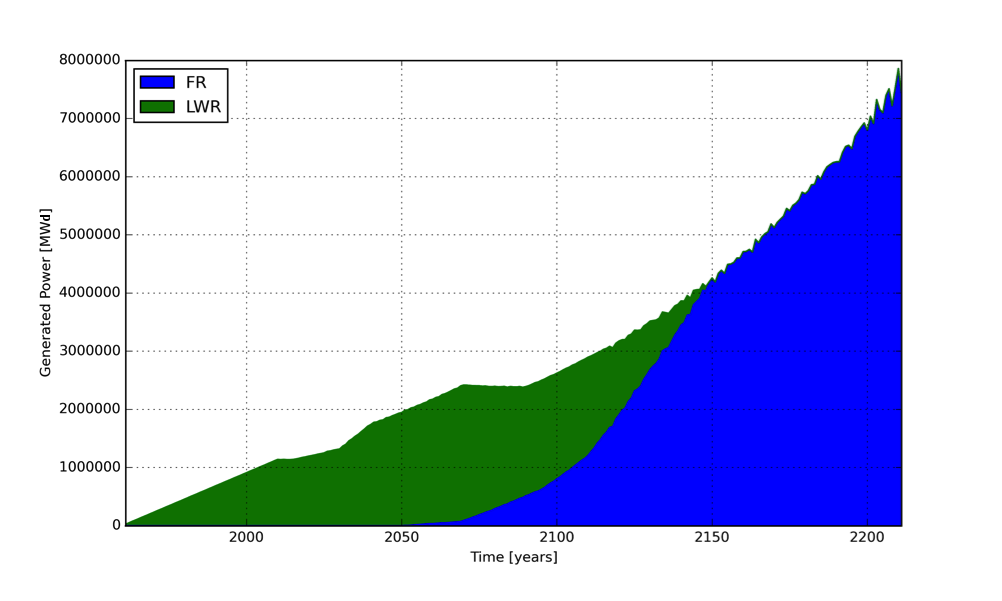Power generated by reactor type.

## Transition Analysis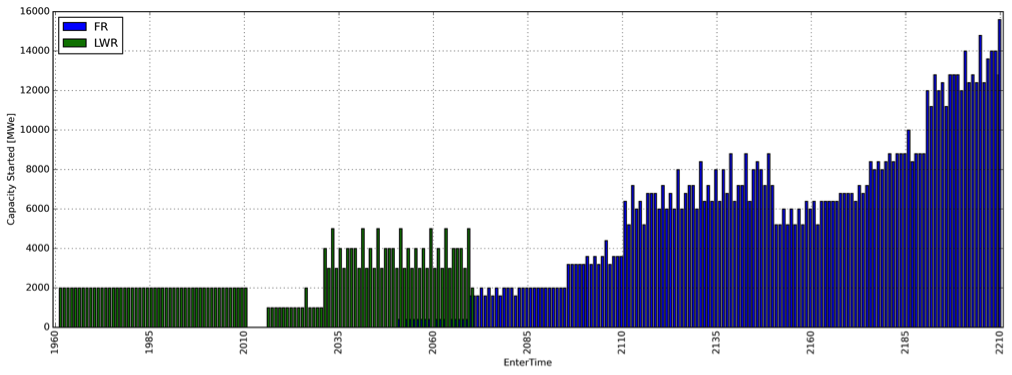Capacity deployed each year, by reactor type.

Canonical Waste Management Comparisons

## Acknowledgements

• Denia Djokic
• Matthew Gidden
• Massimiliano Fratoni
• Ehud Greenspan
• Per Peterson
• Anthony Scopatz
• Xin Wang
• Paul Wilson
• Jasmina Vujic

# THE END

### Katy Huff

katyhuff.github.io/2015-12-08-uiuc